﻿ Numerical Simulation of Effect of Topology on the Airflow Characteristics inside an Indirect Solar DryerPublications are Open
Access in this journal
Article Versions
Export Article
• Normal Style
• MLA Style
• APA Style
• Chicago Style
Research Article
Open Access Peer-reviewed

### Numerical Simulation of Effect of Topology on the Airflow Characteristics inside an Indirect Solar Dryer

DJIAKO Thomas, TAWETSING M. P. Olivier, EDOUN Marcel , DESMORIEUX Helène, KUITCHE Alexis
American Journal of Energy Research. 2018, 6(1), 1-7. DOI: 10.12691/ajer-6-1-1
Published online: June 30, 2018

### Abstract

The present work is part of the optimization of indirect solar dryers. The main objective is to highlight the influence of topological forms of dryers on air settings. For this, we have studied the behavior of air in the collector for four inclinations angles (0°, 30°, 40° and 60°), three values of the height of the drying chamber (1, 1.20 and 1.40 m) and three values of the height of the chimney (0.3, 0.8, and 1.5 m). The Navier-Stokes equations were numerically solved using the finite volume method through the Easy CFD_G package code in its V.4.1.0 version. The numerical simulation was used to define for each load configuration studied, the temperature profile, velocity and pressure. The results show that for the solar collector, 30° is the optimum angle of inclination. This angle provides a better distribution of the velocity vector field. For this angle, the height of the drying chamber allowing a better distribution of velocity and temperature gradient is 1.40 m. The maximum chimney height is 1.5 m. These parameters are used to define an optimal configuration of the indirect solar dryer in which the air temperature varies between 56 and 64°C and drying air velocity between 0.5 and 0.9 m/s.

### 1. Introduction

In an effort to significantly reduce post-harvest losses estimated today at more than 40%, particularly in sub-Saharan Africa 1, several solutions have been proposed including drying. Drying is defined as a method of decreasing the water content of a product by evaporation 2, 3. Among the different types of existing drying, solar drying occupies a special place. This is carried out in dryers called solar dryers. Solar dryers are generally classified according to the heating or the mode of operation into several categories including two main principles: Direct solar dryers and indirect solar dryers 4, 5.

The main objective of a drying operation is to produce a dry product of desired quality (taste, color, texture, nutritional value, etc ....) And at minimum cost 6, he is therefore necessary to optimize its efficiency and performance. The effectiveness of a dryer depends on the characteristics of the product (size, shape, moisture content), the characteristic of the drying air (temperature, relative humidity, velocity) and also topological characteristics of the dryer (size, shape) 7.

Studies have been conducted both on modeling profiles and drying kinetics 8, 9, 10, 11, 12 on improving the efficiency of the dryer 8, 13, 14 and to optimize the drying process. These studies showed that increasing the velocity of the air improves the drying time. Also the influence of temperature on the mass ratio of water evaporated from the product; the higher the temperature, the shorter the drying time. Despite advanced in the literature on drying, challenges in the optimization of its yield still remains to rise.

Several studies have been conducted to optimize the performance of indirect solar dryers on the velocity as well as on the drying time of agro-food products 13, 15, 16, 17, 18, 19. Some stand out as the geometrical characteristics (shape, size) have an influence on the performances of the dryer 7, 20. However, no study put in evidence the influence of the topology (shape) of the dryer on its output or its energetically effectiveness.

The present work enters in the frame work of the optimization of indirect solar dryers with the main objective, highlighting the influence of the shapes of dryers on velocity and drying time of food products, in order to propose the shapes of suitable dryers.

### 2. Material and Methods

Figure 1 presents the basic topological configuration of dryer used in this work.

While leaving therefore this configuration, we proceeded successively by optimization of the various parts of the dryer (the solar collector, the drying chamber and the chimney).

• Figure 1. A basic configuration

Boundary conditions:

Heat flow left again as it follows:

On the solar collector 1000 W/m2;

On the front face of the drying chamber 300W/m2

Structured grid

Temperature of the ambient air: Ta = 27°C;

Turbulent flow: SST

Stationary regime.

Inlet air velocity: Ve = 0.05m/s

2.1. Methodology

Optimization of the angle of the solar collector

We started first by modifying the angle of the collector for four values of α (0°; 30°; 40°; 60°).

Optimization height of the drying chamber

We modified the height of the drying chamber and we used 1 m; 1.2 m and 1.4 m.

Optimization of the chimney

To optimize the chimney the dimensions value according the geometric configuration of figure 2 used are:

H = 0.3; 0.8; 1.5

B= 0.1; 0.4

b= 0.1; 0.3

• Figure 2. Chimney configuration
2.2. Mathematical Model

The numerical simulations are performed using the Reynolds-Averaged Navier-Stokes (RANS) equations. The Boussinesq approximation was used for thermal buoyancy. The Easy CFD_G software package code in its V.4.1.0 version based on the finite volume method for structured and unstructured grids were used to perform the numerical simulations. The discretization scheme used is hybrid for the convective terms in the momentum and energy equations, and the SIMPLEC algorithm for pressure–velocity coupling. For a two dimensional problem, the continuity, momentum and energy equations in the stationary regime are given in a general form by:

• Continuity equation: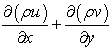(1)

• Momentum conservation equations

Horizontal component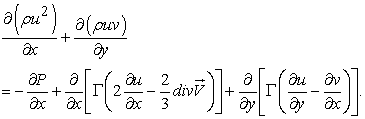(2)

Vertical component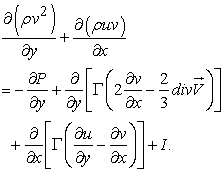(3)

In the previous equations, P (N/m2) represents pressure, k is the turbulence kinetic energy (m2/s2) and I represent the buoyancy forces. The diffusion coefficient is, in this case, given by:(4)

where μ (N s/m2) is the dynamic viscosity and μt is the turbulent viscosity.

• Energy conservation equation

In this case, the dependent variable is the enthalpy Ø = Cp.T(5)

Term ST, in the previous equation, represents the heat generation rate per unit volume (W/m3). The diffusion coefficient is, for the case of a fluid domain: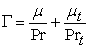(6)

• Turbulence modeling

The properties of a turbulent flow (velocity, pressure, etc.) are not constant in time; instead, they present oscillations about an average value. The numerical calculation of the instantaneous value is not amenable with present day techniques and resources, due to the high temporal and spatial frequencies that characterize these flows. We are, thus, left with the calculation of the average values, only. These can be described through the Reynolds decomposition:(7)

where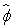is the instantaneous value,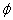is the average value andis the difference between the two (fluctuation). When the Reynolds decomposition is applied to the transport equations and the equations are the averages, some extra terms appear, due to the following property (illustrated for the third term of equation 2):(8)

The last term in the previous equation has the dimensions of a stress and thus, can be expressed as the product of a viscosity (turbulent viscosity) by an average velocity gradient, as in the case of the laminar stresses (this hypothesis was proposed by Boussinesq). The computation of the turbulent viscosity is made recurring to a turbulence model. Easy CFD_G implements the k-ε and the STT (Shear Stress Transport) models. For this study, we used the SST model.

The standard formulation of this turbulence model is described by 21, 22, 23, 24. The turbulent viscosity is given by:(9)

The turbulence kinetic energy, k, as well as its dissipation rate, ε (m2/s3) are computed with the following transport equations:(10)(11)

The term P1 represents the production rate of k as the results of the velocity gradients: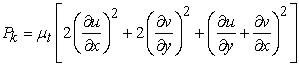(12)

while the term GT accounts for the production or destruction of k and ε due to the thermal gradients: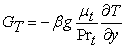(13)

The remaining model constants are: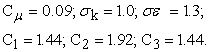### 3. Results and Discussion

Figure 3 shows the meshes of successive configurations studied in order to find the configuration that optimizes the drying parameters of food-products. Our mesh is structured and uniform because we were interested in the behavior of the air throughout the computational domain. Figure 3a shows our starting configuration. Figures 3b and Figures 3c show the first modification of our starting configuration, carried out to obtain the angle of inclination of the collector and the shape of the chimney of the dryer for a better distribution of the velocity and the temperature in the dryer. The figures 3d and 3e show the optimal configurations retained after several changes of the angle of inclination of the collector and the shape of the chimney. They allow us to obtain the best thermal performance by a uniform distribution of temperature and velocity in the dryer.

• Figure 3. Meshes of the studied configurations:α =30°; (b) α = 0°; (c), (d) and (e) α =30°

• Basic configuration

The temperature and velocity fields for the configuration (a) according the Figure 3 are presented by Figure 4. Indeed, in Figure 4a, it is found that the temperature gradually increases as the air passes through the solar collector, the drying chamber and the chimney. The simulation was performed here for an empty dryer (without products), which justifies the high value of the temperature in the chimney. In Figure 4b, the velocity distribution shown that the inside the dryer, the velocity is reaches values of 0.1 m/s and 0.24 m/s. This velocity distribution in the dryer does not guarantee a homogeneous drying and high quality at the end of the operation.

• Figure 4. Temperature field (a) and velocity (b) for the starting configuration
3.1. Optimization of the Angle of the Solar Collector

Figure 5 shows the temperature fields of the first modification of the starting configuration for the four angles of inclination of the solar collector. 5a and 5d show an almost homogeneous distribution of the temperature in the dryer. It is observed in Figure 5a, the high temperatures at the inlet of the collector but which drops considerably at the drying chamber, this is due to the angle of inclination of the collector. For against, these temperatures are relatively low at the input of the collector and increase gradually as the air passes through the dryer for all other configurations. Thus we have temperatures ranging from 36 to 127 °C, 20 to 35 °C and from 33 to 110 °C between the inlet of the collector and the outlet of the dryer respectively for figures 5b, 5c and 5d.

• Figure 5. Temperature distributions of the first modification of the starting configuration

These configurations have shortcomings because, during the simulation we found that the outlet temperature of the dryer decreases over time. This is due to the fact that in addition to natural convection that is to say that operates at low velocity, the height of the chimney does not allow a better bleed air, because it is very close to the drying chamber which is faced to the atmospheric pressure. Over time therefore, the temperature have tendency to be lowered and become uniform throughout the dryer.

• Figure 6. Velocity Field of the first modification of the starting configuration

Figure 6 shows the velocity fields for four angles of inclination of the solar collector. On the Figure 8a, there is a relatively low speed at the inlet of the collector and in the drying chamber, as a result of the angle of inclination of the collector rather the velocity of take-off occurs at the walls of the chamber, is what justifies these high velocities at the walls. By against, for the Figure 8b and 8c, we have very high velocity in the drying chamber because the angle of inclination of the collector ensures a good distribution of the air in the drying chamber. In Figure 6d we have an almost uniform distribution of velocity in the drying chamber, but it is very low because the inclination of the collector is very large. Thus we have velocity that vary from 0.004 to 0.3 m/s; 0.1 to 1.7 m/s; from 0.5 to 1.4 m/s and from 0.001 to 1 m/s to the input of the collector at the outlet of the dryer, respectively to figures 6a, 6b, 6c and 6d. We note at the end of this analysis that, the figure 6b allows us to obtain a homogeneous distribution of velocity and high velocity in the dryer.

Since the optimum angles of inclination of the solar collector is α = 30 °, we optimize the height of the drying chamber to determine if it is possible to obtain better distribution of temperature and velocity in the dryer.

3.2. Optimization of the Drying Chamber

Figure 7 shows the temperature fields for the second modification of the starting configuration. On the Figure 7a, we observe a clear increase in the temperature. At the outlet of the collector we reach a value of 60 °C. In the drying chamber it varies between 61 and 65 °C and reaches 66°C at the level of the chimney. This progressive phenomenon of evolution of the temperature of the solar collector to the chimney is completely normal, more especially as our numerical simulations are done without the products. On the Figure 7b, at the exit of the collector we reach a temperature of 80 °C while in the drying chamber we reach a temperature of 90 °C in the center and 100 °C on the left wall. Moreover we noticed during numerical calculations that after a certain time t, the temperature tends to drop. This phenomenon can be justified by the bad extraction of air which makes the chimney and because of air velocity in natural convection. The height of the chimney being insufficient, this quickly finds itself at the atmospheric pressure.

• Figure 7. Temperature fields relative to height of the drying chamber: a) h = 1.20m, b) h = 1.40m

Figure 8 presents the velocity fields in the configurations relating to the different values of the height of the drying chamber h. On the Figure 8a, at the level of the collector, the velocity of the drying air varies between 0.05 and 0.3 m/s and in the drying chamber is between 0.1 and 0.9 m/s with several dead zones as the figure shows it. While on the Figure 8b, we observe not only an increase but also a good distribution of velocity. In the drying chamber and at the level of the chimney it varies between 0.5 and 3 m/s at the level of the higher left wall it reaches 5.6 m/s.

• Figure 8. Velocity fields: a) h = 1.20 m, b) h = 1.40 m

We see from these results that the increase height of the drying chamber makes it possible to optimize the temperature of the air like its velocity. However it remains a problem, the bleed air into the chamber because it is not rapidly discharged outwardly. We could retain as height of the drying chamber h = 1.40 m. This height provides better results. Thus, we proceed to the optimization of the extraction effect through the chimney.

3.3. Optimization of the Chimney

Considering the optimum angle of the solar collector α = 30° and the height of the drying chamber h = 1.40 m, We increase the height of the chimney for two values of H (0.8 m and 1.5 m) for a fixed base B = 0.40 m in order to find one that will best optimize the performance of the dryer. As shown in Figure 9a, at the collector, the temperature evolves gradually and reaches at exit a value of 140 °C. In the drying chamber, the temperature is between 80 °C and 117 °C. This temperature is very high for the drying of food products. At such temperatures, the products to dry will risk to burning. At the chimney, it varies between 80 °C to 140 °C. These values can be justified that the height of the chimney is not sufficient enough to allow for better extraction. While in Figure 9b, in the collector, there is a gradual evolution of the temperature reaching at its output a value of 100 °C. In the drying chamber, the temperature distribution is quite as satisfactory and is between 56 °C and 64 °C. This temperature range is recommended for drying of various agricultural products. At the chimney, it varies gradually from 82 °C to 124 °C.

• Figure 9. Temperature fields relating to the height of the chimney: a) H = 0.80 m, b) H = 1.5 m.

The following Figure 10 highlights the velocity fields in both configurations. Bad velocity distribution is observed in Figure 10a. Very low velocity is noted throughout the dryer, they vary between 0.3 and 0.6 m/s at the walls of the drying chamber. While on the Figure 10b we observe a good velocity distribution of air drying in the drying chamber. For the inlet air velocity equal to 0.05 m/s, we obtain an average velocity in the dryer equal to 1.01 m/s. So we can keep this last configuration as that allowing achieving good efficiency of the dryer.

Thus, by acting on the three principal parts of the starting dryer, we are succeeded in finding the dryer topology to achieve best drying performance. In addition, while keeping the same simulation conditions, another study on this configuration allows us, by acting on the input and output of the drying chamber, for a height of the drying chamber h = 1.25 m to find a second configuration as well to solve drying problems, this time for lower temperatures (Figure 11). The drying temperature range of food products is generally between 30 °C and 80 °C 16, the second configuration that we propose is in this temperature range with good drying velocity.

• Figure 10. Velocity fields relating to the height of the chimney: a) H = 0.80 m, b) H = 1.5 m.

Figure 11 shows the final configuration temperature range studied this time with h = 1.25 m. The temperature in this dryer is between 53 and 56.7 °C. It evolves gradually and becomes almost constant at around 55 °C inside the drying chamber.

• Figure 11. Temperature fields for h = 1.25m

Figure 12 shows the velocity field for this last configuration. The velocity distribution in this figure is quite as satisfactory as the previous one. This varies between 0.5 and 0.9 m/s.

• Figure 12. Velocity fields for h = 1.25 m

In the end, we get two topological dryer configurations which can help to optimize the drying of food products. We present as a summary the results of the two selected configurations (Figure 13).

• Figure 13. Topological configurations retained

These configurations as shown in these figures, allow us to obtain good drying parameters such as air velocity and temperature. Thus, we obtain for an air velocity in entry of the dryer of 0.05 m/s in natural convection and an ambient temperature of 27 °C, the following values:

For the configuration of Figure 13a, a drying air velocity is between 0.3 and 1.3 m/s at the center of the dryer, a drying temperature between 56 and 64°C. A drying air velocity between 0.5 and 0.9 m/s at the center of the dryer and a drying temperature between 53 and 56.7°C for the configuration of Figure 13b. These intervals of temperatures are recommended for the drying of several food-products 1.

### 4. Conclusion

In this work, it was question to highlight the influence of the topological configuration of indirect solar dryers on the temperatures, velocity and drying times of food products. The analyses of the temperature and velocity fields for all configurations studied by numerical simulation have allowed us to achieve two geometrical configurations of indirect solar dryer to optimize the efficiency of the dryers. The results show indeed that the geometrical shapes of the dryer have a very significant influence on the drying parameters. As a perspective, the results of this work is purely theoretical, we plan to do a practical study through the physical realization of the dryer. Moreover simulations having been made without de product, we will do another study this time, taking into account the mass transfer and material between the product and air. In perspective, it would be interesting to associate in this study, the transfer of mass and matter between the product and air, in order to analyze their influence on the various parameters of drying such as velocity and temperatures drying.

### Nomenclature

Cp: constant pressure specific heat (J/kg K)

H: height (m)

H: height (m)

Øconv: thermal power exchanged by convection

P: pressure (N/m2)

T: temperature (°C)

T: time (s)

St: heat generation rate per unit volume (W/m3)

u, v: velocities in x and y direction (m/s)

x, y: coordinates (m)

k: turbulence kinetic energy (m2/s2)

I: buoyancy forces

Pr: Prandtl number

U: (m/s)

### Greek Symbols

μ: dynamic viscosity (N s/m2)

Γ: diffusion coefficient

ρ: density (kg/m3)

ε: dissipation rate (m2/s3)

### References

  Edoun M., Kuitche A., Marouzé C., Giroux F., Kapseu C. (2010). Etat de la pratique du séchage de quelques fruits et légumes à petite échelle dans le Sud Cameroun. Fruits Cirad/EDP Sciences (1), pp. 25-36. In article  Mujumdar A. S. (1997). modeling and numerical simulation Solar drying foods, Solar Energy, N° 314, pp. 151-157. In article  Bimbenet J. J., Duquenoy A., Trystram G. (2002). Génie des Procédés alimentaires. Des bases aux applications, Dunod, Paris. In article  Daguenet M. (1985). Les Séchoirs solaires : théorie et pratique. Unesco. In article  Ekechukwu O.V., Norton B. (1999). Review of solar-energy drying systems II: an overview of solar drying technology, Energy Conversion & Management, Vol 40, pp. 615-655. In article View Article  Edoun M., Takamte G., Monkam L., Kuitche A., Kamga R. (2013). Numerical Simulation of Convective Drying of Mangoes (mangiferaIndica L.) Under Variable Thermal Conditions, INPRESSCO International Journal of Thermal Technologies, Vol 3 (2), pp. 48-52. In article View Article  Sokhansanj S., Jayas D. (1995). Drying of Foodstuffs”, In: Mujumdar A.S., Editor. Handbook of Industrial Drying, 2. New York: Marcel Decker Inc. In article  Kouhila M., Belghit A., Kaoua M., Daguenet M. (1998). Etude Expérimentale du Séchage Convectif de la Menthe dans un Séchoir Modulaire Polyvalent à Chauffage partiellement Solaire Revue des Energies Renouvelables, pp.1-5. In article View Article  Edoun M., Fokone A. T., Kuitche A., Kengne C. (2013). Modeling of Drying Kinetics of Fresh Carrot (Daucus carota), International Journal of Innovation and Applied Studies, Vol4 (2), pp. 375-381. In article  Kuitche A., Kouam J., Edoun M. (2006). Modélisation du profil de température dans un séchoir construit dans l’environnement tropical, Journal of Food Engineering, Vol76, pp. 605-610. In article View Article  Kuitche A., Edoun M., Takamte G.. ‘2007). Influence of pretreatment on the drying kinetic of a local Okro (Hibiscus esculentus) Variety, World Journal of Dairy and Food Sciences, Vol 2 (2), pp. 83-88. In article View Article  Mokretar Sofiane (2009). Contribution théorique et expérimentale à l’étude du bilan d’énergie et de masse d’un séchoir solaire de type serre. Application à la détermination de la cinétique de séchage des prunes”, Thèse de Doctorat, Université des Sciences et de la Technologie. In article  Dadda B., Kherrour S., Serir L. (2008). Réalisation d’un séchoir solaire indirect, Revue des Energies Renouvelables, pp. 127-134. In article  Cristiana B. M., Guimarães A. F., L. C-G., S. de Hanriot M., T. de Martins O. (2012). Simulation of the airflow inside a hybrid dryer, IJRRAS, Vol10 (3), pp. 382-389. In article View Article  Abdelhamid B. (1997). Etude numérique d’un séchoir solaire fonctionnant en convection forcée. Revue générale de thermique, Vol 36, pp. 837-850. In article View Article  Miri R., Mokrani O., Siad F., Belhamel M. (2002). Etude Expérimentale d’un Séchoir Solaire, Revue des Energies Renouvelables, pp. 41- 48. In article  Boubeghal A., Benhammou M., Moungar H. (2008). Conception et simulation numérique d’un séchoir solaire à convection Forcée: Application sur le séchage de piment, 2ème séminaire maghrébin sur les sciences et les technologies de séchage SMSTS. In article  Heilporn (2012). Contribution au développement d’une nouvelle technologie de séchage solaire. application à la mangue. Thèse de Doctorat, Université libre de Bruxelles (ULB), École polytechnique de Bruxelles. In article View Article  Andrew R. H. R., Jakhrani A. Q., Kamboh S. A. Tiong Kie P. L. (2013). Development of an Indirect Solar Dryer with Biomass Backup Burner for Drying Pepper Berries, World Applied Sciences Journal, Vol22 (9), pp. 1241-1251. In article View Article  Augustus L. M, Kumar S., Bhattacharya S.C. (2002). A comprehensive procedure for performance evaluation of solar food dryers, Renewable and Sustainable Energy Reviews, Vol 6, pp. 367-393. In article View Article  Gameiro Lopes A. M..(2012). Easy CFD_G V4.1.0 User’s Manual. In article  Launder B.E.., Spalding D.B. (1972). Mathematical models of turbulence. Academic Press London and New York, ISBN 0-12-438050-6. In article PubMed  Launder B.E., Spalding D.B. (1974). The numerical computer of Turbulent Flows, Computer Methods in Applied Mechanics and Engineering, Vol3, pp. 269-289. In article View Article  Djilali N., Gantshore I.., Salcudean M. (1987). Calculation of convective heat transfer in recirculating turbulent flows using various Near-wall turbulence models, Numerical Heat Transfer, 16, pp. 189-212. In article View Article

Published with license by Science and Education Publishing, Copyright © 2018 DJIAKO Thomas, TAWETSING M. P. Olivier, EDOUN Marcel, DESMORIEUX Helène and KUITCHE AlexisThis work is licensed under a Creative Commons Attribution 4.0 International License. To view a copy of this license, visit http://creativecommons.org/licenses/by/4.0/